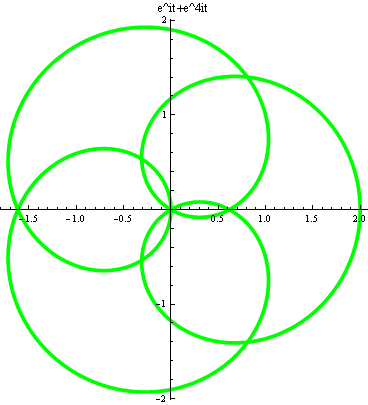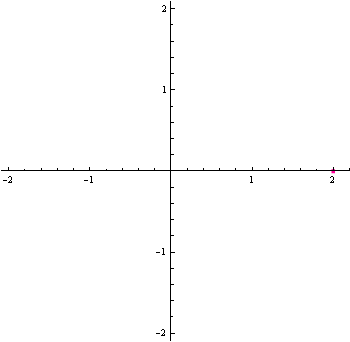## Examples in complex analysis (includes a MATLAB and MATHEMATICA example))

Example 1: Compute the integral
$$\int_{\gamma}\frac{z^3+1}{z^3-1}dz,$$
where $$\gamma$$ is the curve $$z=e^{it}+e^{4it},t\in[0,2\pi]$$.

Hint:

The picture of the curve is shown as followsThe animation below shows the process from t=0 to $$2\pi$$:You do not have to plug in $$z=e^{it}+e^{4it},t\in[0,2\pi]$$ in order to solve this problem.

Example 2: Evaluate the contour integral
$$\int_{\gamma}\frac{1}{e^z-1}dz,$$
where $$\gamma$$ is the circle with radius $$3\pi$$, centered at $$z=0$$, oriented counterclockwise for one loop.
Solution 1: There are 3 singularities inside the circle: $$0, \pm 2\pi i$$. All of them has residue $$2\pi i$$, so the answer is $$6\pi i$$ by the residue theorem.

Solution 2: Using Matlab, the code is:

Solution 3: Using Mathematica.  $$N\left[3 \pi \int_0^{2 \pi } \frac{i e^{i x}}{-1+e^{3 \pi e^{i x}}} \, dx\right]$$ gives an answer: $$0.\, +12.5664 i$$. Is this correct? What's wrong?